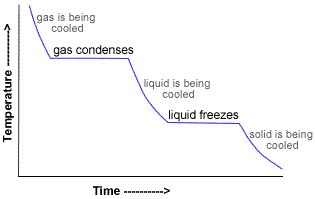# Calculate the number of joules given off when 32.0 grams of steam cools from 110.0 °C to ice at -40.0 °C.

May 29, 2014

The amount of energy given off is 99 600 J.

#### Explanation:

There are five heats to consider:${q}_{1}$ = heat lost on cooling steam from 110.0 °C to 100 °C.

${q}_{2}$ = heat lost on condensing steam to water at 100 °C.

${q}_{3}$ = heat lost on cooling water from 100 °C to 0°C.

${q}_{4}$ = heat lost on freezing water to ice at 0 °C.

${q}_{5}$ = heat lost on cooling ice from 0 °C to -40.0 °C.

The total heat evolved is

$q = {q}_{1} + {q}_{2} + {q}_{3} + {q}_{4} + {q}_{5}$

1. Cooling the Steam

$m = \text{32.0 g H"_2"O}$

For steam, the specific heat capacity, $c = \text{2.010 J·g"^"-1""°C"^"-1}$.

ΔT = T_2 – T_1 = "(100.0 - 110.0) °C" = "-10.0 °C"

q_1 = mcΔT = 32.0 color(red)(cancel(color(black)("g"))) × "2.010 J·"color(red)(cancel(color(black)( "°C"^"-1""g"^"-1"))) × ("-10.0" color(red)(cancel(color(black)("°C")))) = "-643 J"

2. Condensing the Steam

$\text{Heat of condensation = -Heat of vaporization}$

Δ H_"cond" = ""-ΔH_"vap" = "-2260 J·g"^"-1"

q_2 = m Δ H_"cond" = 32.0 color(red)(cancel(color(black)("g")))×("-2260 J·"color(red)(cancel(color(black)("g"^"-1")))) = "-72 320 J"

3. Cooling the Water

For liquid water, the specific heat capacity, $c = \text{4.184 J·°C"^"-1""g"^"-1}$.

ΔT = T_2 – T_1 = "(0 - 100) °C" = "-100 °C"

q_3 = mcΔT = 32.0 color(red)(cancel(color(black)("g"))) × "4.184 J·"color(red)(cancel(color(black)("°C"^"-1""g"^"-1")))× ("-100"color(red)(cancel(color(black)("°C")))) = "-13 389 J"

4. Freezing the Water

$\text{Heat of freezing = -Heat of fusion}$

$\text{-"ΔH_"fus" = "334 J·g"^"-1}$

ΔH_"freeze" = "-"ΔH_"fus" = "-334 J·g"^-1"

${q}_{4} = \text{-"m Δ H_"fus" = 32.0 color(red)(cancel(color(black)("g"))) × "-334 J·"color(red)(cancel(color(black)("g"^"-1"))) = "-10 689 J}$

5. Cooling the Ice

The specific heat capacity of ice, $c = \text{2.03 J·°C"^"-1""g"^"-1}$

ΔT = T_2 – T_1 = "(-40.0 - 0) °C" = "-40.0 °C"

q_5 = mcΔT = 32.0 color(red)(cancel(color(black)("g"))) × 2.03 "J·"color(red)(cancel(color(black)("°C"^"-1""g"^"-1"))) × (color(red)(cancel(color(black)("-40.0 °C")))) = "-2598 J"

Adding them all up

$q = {q}_{1} + {q}_{2} + {q}_{3} + {q}_{4} + {q}_{5} = \text{(-643 – 72 320 – 13 389 – 10 689 - 2598) J" = "-99 600 J}$